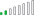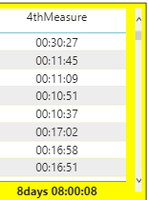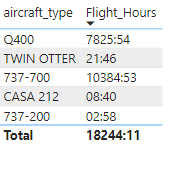cancel
Showing results for
Did you mean:Resolver II

## Sum of H:MM:SS

Hello Everyone,

I am new to Power BI and I am trying to figure out this problem. I have a field called [talk time] in Excel that is H:MM SS format. I brought the data into to Power BI and changed the format to HH:MM SS. What I am trying to do is sum the [talk time] data. When I just use the DAX of “SumTalkTime =Sum(TableName[talk time])” then I get 4:45:01 when it should be 28:45:01. How do I get the Power BI to show the correct sum in the HH:MM SS format?

Once I get that done I have more columns that are the same format. If I wanted to add [SumTalkTime] to another summed column let’s call it [SumTalk2], would I just use =Sum(SumTalkTime+ SumTalk2)”

As I looked through the forum, I tried to sum the talk time using just the normal sum function. Then I tried to go back to change it to the time format I need using the DAX formula below and I get a conversion error.

RevisedTT = (LEFT('Agent State Summary'[SumTalkTime],FIND(":",'Agent State Summary'[SumTalkTime])-1)*60+RIGHT('Agent State Summary'[SumTalkTime],LEN('Agent State Summary'[SumTalkTime])-FIND(":",'Agent State Summary'[SumTalkTime]))*1)/60

Cannot convert Value ‘12/31/1899 12’ to type text to type number

Here is some example data

 Talk Time Talk 2 TOTAL 4:11:37 4:11:37 8:23:14 3:41:17 3:41:17 7:22:34 4:02:22 4:02:22 8:04:44 5:24:39 5:24:39 10:49:18 3:47:59 3:47:59 7:35:58 2:54:36 2:54:36 5:49:12 4:42:31 4:42:31 9:25:02 28:45:01 28:45:01 57:30:02
1 ACCEPTED SOLUTIONResolver II

Thank you for the responses,

I have tried a DAX formula that seems to work.

SumTalkTime =

VAR TotalSeconds=SUMX('Table Name',HOUR('Table Name'[Column])*3600+MINUTE('Table Name'[Column])*60+SECOND('Table Name'[Column]))
VAR Days =TRUNC(TotalSeconds/3600/24)
VAR Hors = TRUNC((TotalSeconds-Days*3600*24)/3600)
VAR Mins =TRUNC(MOD(TotalSeconds,3600)/60)
VAR Secs = MOD(TotalSeconds,60)
return IF(DAYS=0,"",IF(DAYS>1,DAYS&"days ",Days&"day"))&IF(Hors<10,"0"&Hors,Hors)&":"&IF(Mins<10,"0"&Mins,Mins)&":"&IF(Secs<10,"0"&Secs,Secs)

19 REPLIES 19Frequent Visitor

Also very new to Power Bi and feeling really out my depth tbh.

I'm looking for something very similair to this with one addition, I want a total per month / day, not just a grand total.

I've attempted the new measures (quick measures) and they just seem to do a CountA rather than doing a SUM of the data.

Any help would be appreciated and in Dummy terms 😀Resolver II

Not knowing what data you have, I would say the easiest thing to do is to do all your calculations in seconds and then convert from Seconds to time. That has been what works for me. Based on what you are looking for, you want to do a matrix visual and have Month / Date as your rows and the Format(sum(time in seconds) / 86400, "HH:MM:SS"). Power BI has some good tutorials on the basics of visuals, but there are a ton of Youtube videos out there.

hope this helps

Have fun

Welcome to Power BIAnonymous
Not applicable

Hi @Jorgast ,

I used the DAX as suggested in this post to solve my issue with the duration. However, in the table or matrix visualization when I select the total duration in ascending/descending order, the highest value duration comes in between other columns instead of at the end(ascending) or at the start (descending). Any idea how to place this value in the proper sorting order?Total Duration =
VAR TotalSeconds= SUMX('Total calls-Monthly',HOUR('Total calls-Monthly'[Duration])*3600+MINUTE('Total calls-Monthly'[Duration])*60+SECOND('Total calls-Monthly'[Duration]))
VAR Days =TRUNC(TotalSeconds/3600/24)
VAR Hors =TRUNC((TotalSeconds-Days*3600*24)/3600)
VAR Mins =TRUNC(MOD(TotalSeconds,3600)/60)
VAR Secs =MOD(TotalSeconds,60)
return IF((Hors + (Days*24))<10,"0"&(Hors + (Days*24)),(Hors + (Days*24)))&":"&IF(Mins<10,"0"&Mins,Mins)&":"&IF(Secs<10,"0"&Secs,Secs)Resolver II

@Anonymous

If you have a total seconds column, that would probably be the easiest thing.Helper I

How can I convert the days return to show only in hours: minutes: seconds.  Basically convert my 8 days to hours?

I want it to show - 200:00:084thMeasure = VAR TotalSeconds= SUMX('SAP Time Allocations',HOUR('SAP Time Allocations'[2ndMeasure])*3600+MINUTE('SAP Time Allocations'[2ndMeasure])*60+SECOND('SAP Time Allocations'[2ndMeasure]))
/*HOUR('SAP Time Allocations'[SecondMeasure])*3600 + MINUTE('SAP Time Allocations'[SecondMeasure])*60+SECOND('SAP Time Allocations'[SecondMeasure])*/

VAR Days =TRUNC(TotalSeconds/3600/24)
VAR Hours = TRUNC((TotalSeconds-Days*3600*24)/3600)
VAR Mins =TRUNC(MOD(TotalSeconds,3600)/60)
VAR Secs = MOD(TotalSeconds,60)
return IF(DAYS=0,"",IF(DAYS>1,DAYS&"days ",Days&"day"))&IF(Hours<10,"0"&Hours,Hours)&":"&IF(Mins<10,"0"&Mins,Mins)&":"&IF(Secs<10,"0"&Secs,Secs)Resolver II

Thank you for the responses,

I have tried a DAX formula that seems to work.

SumTalkTime =

VAR TotalSeconds=SUMX('Table Name',HOUR('Table Name'[Column])*3600+MINUTE('Table Name'[Column])*60+SECOND('Table Name'[Column]))
VAR Days =TRUNC(TotalSeconds/3600/24)
VAR Hors = TRUNC((TotalSeconds-Days*3600*24)/3600)
VAR Mins =TRUNC(MOD(TotalSeconds,3600)/60)
VAR Secs = MOD(TotalSeconds,60)
return IF(DAYS=0,"",IF(DAYS>1,DAYS&"days ",Days&"day"))&IF(Hors<10,"0"&Hors,Hors)&":"&IF(Mins<10,"0"&Mins,Mins)&":"&IF(Secs<10,"0"&Secs,Secs)Regular Visitor

New to powerbi and really appreciate this formula. Is there a way show empty values as null/blank instead of 0:0 to not bring them in? Currently I just filter show values that do not contain 0:0 additionally, the sort does not show correctly when using this method?Resolver II

I would probably add the ISBLANK / ISERROR function before the if statement since you want to catch blank/null data. You can also filter the data out in your visual as well. As for the sorting, I did not intend on sorting the data for this report is was working on. Without knowing your data model, you may want to look at sorting based on the sum of seconds.Regular Visitor

Thanks @Jorgast

Appreciate it, worked as needed.

For anyone else who may need the formula, I used:

``````SumofTime =
VAR TotalSeconds = SUMX('YourTable', HOUR('YourTable'[Column1]) * 3600 + MINUTE('YourTable'[Column1]) * 60 + SECOND('YourTable'[Column1]))
VAR Days = TRUNC(TotalSeconds / 3600 / 24)
VAR Hours = TRUNC((TotalSeconds - Days * 3600 * 24) / 3600)
VAR Minutes = TRUNC(MOD(TotalSeconds, 3600) / 60)
VAR Seconds = MOD(TotalSeconds, 60)
RETURN
IF(
ISBLANK('YOURTABLE'[NewMeasureofSumfromColumn1]),
BLANK(),
IF(
(Hours + (Days * 24)) < 10,
"0" & (Hours + (Days * 24)),
(Hours + (Days * 24))
)
& ":" &
IF(
Minutes < 10,
"0" & Minutes,
Minutes
)
)``````New Member

I have a short date field, which should be at filter for my calculation.

How should i incorporate it ?New Member

Hi!

could you please tell me what is your data type in this column?Resolver II

For me, the data came in seconds as a whole number.Helper III

Short and easy, well done
Thank you 🙂Helper III

Short and easy, well done
Thank you @Jorgast  🙂Helper I

I have similar situation but with one change. One of the columns has negative value in it. Your formula did work for 2 columns but gave an error for the negative value column. Any idea how to fix this?Resolver II

@nikhilmanohar

I know this may sound very basic, but i would just create a seperate column that checks for negative values. Something like -IF(Table[Column] < 0, Table[Column]*-1, Table[Column])Anonymous
Not applicable

Power BI doesn't handle Duration as a data type right now.  See this post for some discussion and sample DAX (for AVERAGE rather than SUM) that handles imported durations: https://community.powerbi.com/t5/Desktop/Format-the-average-of-a-duration/td-p/95322

And please vote to get this improved here: https://ideas.powerbi.com/forums/265200-power-bi-ideas/suggestions/8814178-field-of-duration-typeYou can do this in Power Query (Get Data) by adding a custom column and converting your time into decimal hours

= Time.Hour([TimeColumn]) + Time.Minute([TimeColumn])/24)

* Matt is a Microsoft MVP (Power BI) and author of the Power BI Book Supercharge Power BI.Anonymous
Not applicable

hi, thank you for this idea, just to inform you to get the correct calculation of time you have to divide minutes by 60 and then you will get the proper decimal numbers ie. = Time.Hour([TimeColumn]) + Time.Minute([TimeColumn])/60)